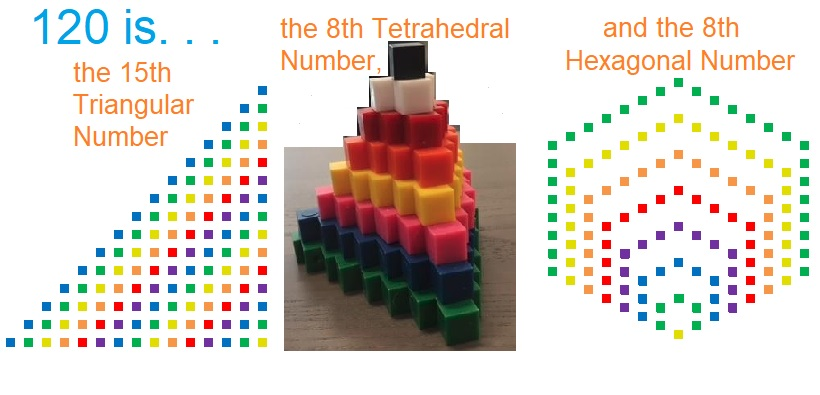# 120 and Level 5

Contents

### Today’s Puzzle:

Write the numbers from 1 to 10 in both the top row and the first column so that this puzzle functions like a multiplication table.

### Thinking process using divisibility rules to find the factor pairs of 120:

√120 is irrational and approximately equal to 10.95. Every factor pair of 120 will have one factor less than 10.95 and one factor greater than 10.95, and we will find both factors in each pair at the same time. The following numbers are less than 10.95. Are they factors of 120?

1. Yes, all whole numbers are divisible by 1, so 1 x 120 = 120.
2. Yes, 120 is an even number. 120 ÷ 2 = 60, so 2 x 60 = 120. (Since 60 is even, 4 will also be a factor of 120.)
3. Yes, 1 + 2 + 0 = 3 which is divisible by 3 (but not by 9), so 120 is divisible by 3. 120 ÷ 3 = 40, so 3 x 40 = 120. Note 120 will not be divisible by 9.
4. Yes, the number formed from its last two digits, 20, is divisible by 4, so 120 is divisible by 4, and 4 x 30 = 120. (Note since 30 is even, 8 will also be a factor of 120.)
5. Yes, the last digit is 0 or 5, so 120 is divisible by 5, and 5 x 24 = 120.
6. Yes, 120 is divisible by both 2 and 3, so it is divisible by 6, and 6 x 20 = 120.
7. No. The divisibility trick for 7 requires us to split 120 into 12 and 0. We double 0 and subtract the double from 12. 12 – (2 x 0) = 12 – 0 = 12. Since 12 is not divisible by 7, 120 also is not divisible by 7.
8. Yes, see 4 above. 120 = 8 x 15. (This will mean that ANY number whose last 3 digits are 120 will also be divisible by 8.)
9. No, see 3 above. 120 is not divisible by 9.
10. Yes, 120 ends with a zero, so 10 is a factor of 120, and 10 x 12 = 120.

From this thinking process we conclude that the factor pairs of 120 are 1 x 120, 2 x 60, 3 x 40, 4 x 30, 5 x 24, 6 x 20, 8 x 15, and 10 x 12.

### Factors of 120:

120  is a composite number. 120 = 1 x 120, 2 x 60, 3 x 40, 4 x 30, 5 x 24, 6 x 20, 8 x 15, or 10 x 12. Factors of 120: 1, 2, 3, 4, 5, 6, 8, 10, 12, 15, 20, 24, 30, 40, 60, 120. Prime factorization: 120 = 2 x 2 x 2 x 3 x 5, which can also be written 120 = 2³ x 3 x 5.When 120 is a clue in the FIND THE FACTORS 1 – 12 puzzles, use 10 and 12 as the factors.

### Sum-Difference Puzzle:

30 has four factor pairs. One of those factor pairs adds up to 13, and another one subtracts to 13. Can you write those factors in their proper places in the first puzzle below?

120 has eight factor pairs. One of those factor pairs adds up to 26, and another one subtracts to 26. If you can identify those factor pairs, then you can solve the second puzzle!The second puzzle is really just the first puzzle in disguise. Why would I say that?

### More about the Number 120:

120 = 5! because 1·2·3·4·5 = 120

120 is also the smallest positive multiple of 6 that is neither preceded nor followed by a prime number! (119 = 7 ×1 7, and 121 = 11 × 11, so neither one is prime.)

What kind of shape is 120 in?

• 120 is the 15th triangular number because 15(16)/2 = 120,
it’s the 8th tetrahedral number because (8)(9)(10)/6 = 120 (That means
• 120 is the sum of the first eight triangular numbers), and
• it is the 8th hexagonal number because (8)(2·8-1) = 120.120 is the hypotenuse of a Pythagorean triple:
72-96-120, which is 24 times (3-4-5).

### A Logical Way to Find the Solution to Today’s Puzzle:## 6 thoughts on “120 and Level 5”

1.Steve Morris

You are making numbers very interesting!

•ivasallay

Thank you. If numbers are interesting or boring is always a personal decision. I like the decision you’ve made!

•Joseph Nebus

Oh, I like that attitude. There is almost always something interesting to be found in numbers, anyway.

2.ivasallay

So true. I’ve decided to add a link to each post with interesting facts about the factored number.

•Steve Morris

yay!

•Joseph Nebus

Yeah, the little facts are fun extras.

This site uses Akismet to reduce spam. Learn how your comment data is processed.